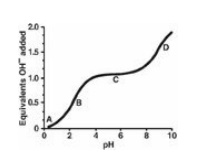# Study Questions and Answers, Chapter Summary

| Home | | Biochemistry |

## Chapter: Biochemistry : Amino Acids

Choose the ONE best answer, Study Questions and Answers, Chapter Summary - Amino Acids - Biochemistry

CHAPTER SUMMARY

Each amino acid has an α-carboxyl group and a primary α-amino group (except for proline, which has a secondary amino group). At physiologic pH, the α-carboxyl group is dissociated, forming the negatively charged carboxylate ion (– ICOO–), and the α-amino group is protonated (– INH3+). Each amino acid also contains one of 20 distinctive side chains attached to the α-carbon atom. The chemical nature of this R group determines the function of an amino acid in a protein and provides the basis for classification of the amino acids as nonpolar, uncharged polar, acidic (polar negative), or basic (polar positive). All free amino acids, plus charged amino acids in peptide chains, can serve as buffers. The quantitative relationship between the pH of a solution and the concentration of a weak acid (HA) and its conjugate base (A-) is described by the Henderson-Hasselbalch equation. Buffering occurs within ±1 pH unit of the pKa and is maximal when pH = pKa, at which [A-] = [HA]. The α-carbon of each amino acid (except glycine) is attached to four different chemical groups and is, therefore, a chiral, or optically active carbon atom. The L-form of amino acids is found in proteins synthesized by the human body.

Study Questions

1.1 Which one of the following statements concerning the titration curve for a nonpolar amino acid is correct? The letters A through D designate certain regions on the curve below.A. Point A represents the region where the amino acid is deprotonated.

B. Point B represents a region of minimal buffering.

C. Point C represents the region where the net charge on the amino acid is zero.

D. Point D represents the pK of the amino acid’s carboxyl group.

E. The amino acid could be lysine.

Correct answer = C. C represents the isoelectric point, or pI, and as such is midway between pK1 and pK2 for a nonpolar amino acid. The amino acid is fully protonated at Point A. Point B represents a region of maximum buffering, as does Point D. Lysine is a basic amino acid, and has an ionizable side chain.

1.2 Which one of the following statements concerning the peptide shown below is correct?

Val-Cys-Glu-Ser-Asp-Arg-Cys

A. The peptide contains asparagine.

B. The peptide contains a side chain with a secondary amino group.

C. The peptide contains a side chain that can be phosphorylated.

D. The peptide cannot form an internal disulfide bond.

E. The peptide would move to the cathode (negative electrode) during electrophoresis at pH 5.

Correct answer = C. The hydroxyl group of serine can accept a phosphate group. Asp is aspartate. Proline contains a secondary amino group. The two cysteine residues can, under oxidizing conditions, form a disulfide (covalent) bond. The net charge on the peptide at pH 5 is negative, and it would move to the anode.

1.3 A 2-year-old child presents with metabolic acidosis after ingesting an unknown number of flavored aspirin tablets. At presentation, her blood pH was 7.0. Given that the pKa of aspirin (salicylic acid) is 3, calculate the ratio of its ionized to un-ionized forms at pH 7.0.

Correct answer = 10,000 to 1.

pH = pKa + log [A-]/[HA]. Therefore, 7 = 3 + × and × = 4. The ratio of A- (ionized) to HA (un-ionized), then, is 10,000 to 1 because the log of 10,000 is 4.